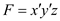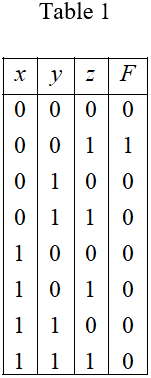Problem

# Solutions For Digital Design Chapter 2 Problem 2PE

#### Step-by-Step Solution

Solution 1

Consider the following Boolean expression:

$$F=x^{\prime} y^{\prime} z$$

Observe that there are three variables $$x, y$$, and $$z$$, hence three variable truth table is required.

Design a truth table for the expression, with F as output.

The following table shows the truth table of the function,.Note that the function $$F$$ is high only when $$x=0, y=0$$, and $$z=1$$, because the function is AND operation of negation of $$x$$, negation of $$y$$ with $$z$$ that is, $$F=x^{\prime} y^{\prime} z$$.

Therefore, the truth table for the Boolean expression is shown in Table 1 .